Electron. J. Diff. Equ., Vol. 2011 (2011), No. 10, pp. 1-10.

### Vector-valued Morrey's embedding theorem and Holder continuity in parabolic problems Patrick J. Rabier

Abstract:
If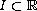is an open interval and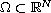an open subset with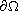Lipschitz continuous, we show that the space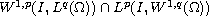is continuously embedded in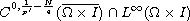if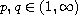and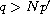. When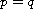, this coincides with Morrey's embedding theorem for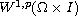. While weaker results have been obtained by various methods, including very technical ones, the proof given here follows that of Morrey's theorem in the scalar case and relies only on widely known properties of the classical Sobolev spaces and of the Bochner integral.
This embedding is useful to formulate nonlinear evolution problems as functional equations, but it has other applications. As an example, we derive apparently new space-time Holder continuity properties for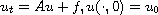when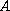generates a holomorphic semigroup on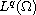.

Submitted January 11, 2011. Published January 19, 2011.
Math Subject Classifications: 46E35, 46E40, 35K90, 35K55.
Key Words: Morrey's theorem; embedding; vector-valued Sobolev space; mixed norm.

Show me the PDF file (264 KB), TEX file, and other files for this article.Patrick J. Rabier Department of Mathematics, University of Pittsburgh Pittsburgh, PA 15260, USA email: rabier@imap.pitt.edu